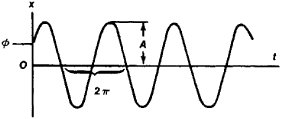# Harmonic Oscillations

The following article is from The Great Soviet Encyclopedia (1979). It might be outdated or ideologically biased.

## Harmonic Oscillations

oscillations during which a physical quantity varies with time according to a sine or cosine law. Harmonic oscillations are represented graphically by a sine or cosine curve (see Figure 1); they can be written in the form x = A sin(ωt + φ) or x = A cos(ω + φ), where x is the value of the oscillating quantity at time t (for mechanical harmonic oscillations, for example, the displacement or velocity; for electrical harmonic oscillations, voltage or current strength), A is the amplitude of the oscillation, ω is the angular frequency, (ωt + φ) is the phase of the oscillation, and φ is the initial phase of the oscillation.Figure 1. Harmonic oscillations

Harmonic oscillations occupy an important place among the various types of oscillations; this is determined by two circumstances. First, oscillatory processes whose form is close to harmonic oscillations are often encountered in nature and technology. Second, a very broad class of systems whose properties can be considered invariable (for example, electrical circuits in which the inductance, capacitance, and resistance do not depend on the voltage or current strength) behave in a special way in relation to harmonic oscillations: when acted upon by harmonic oscillations, the induced oscillations they perform are also harmonic oscillations. (When the form of the external action is not harmonic oscillation, the system’s induced oscillations always differ from the form of the external action.) In other words, in most cases harmonic oscillations are the only type of oscillation whose form is not distorted upon reproduction; this fact determines the special value of harmonic oscillations, as well as the possibility of representing nonharmonic oscillations in the form of a harmonic spectrum of oscillations.

### REFERENCES

Elementarnyi uchebnik fiziki, 3rd ed., vol. 3. Edited by G. S. Landsberg. Moscow, 1962.
Khaikin, S. E. Fizicheskie osnovy mekhaniki. Moscow, 1963.
References in periodicals archive ?
Already in 1950 Gantmacher and Krein  have demonstrated that continued fractions are solutions of the Euler-Lagrange equation for low amplitude harmonic oscillations in simple chain systems.
Harmonic oscillations of heterogeneous magneto-electroelastic cylinders were studied .
It should be noted that, depending on sources of distortion, a significant spectrum of interharmonics (IG) can be generated along with the HG, which, in accordance with the IEC standard, include harmonic oscillations with frequencies not multiples of the frequency of the supply network .
To define a repeatable and comparable basis for gust generation this research focuses on harmonic oscillations of the flow direction in the range of the test section of a wind tunnel.
They consist mainly of quasi harmonic oscillations with variable phases and amplitudes and small irregular variations, containing abrupt changes due to certain natural sources of impulse excitations.
Coupling the inter-site Morse potential to the on-site Morse potential offers a variety of possibilities as the latter favours the onset of DB (for the case of on-site harmonic oscillations coupled to inter-site harmonic oscillations see  and references therein).
In this case harmonic oscillations are excited in frequency range from 0 Hz at [[omega].sub.2] = [[omega].sub.1], A = [A.sub.max].
It is noteworthy that similar phenomenon of second harmonic oscillations at DC bias was also observed in GaN based SSDs using an entirely 2D model .
We soon discovered carbon recovered from harmonic oscillations and paradox flex faster than aluminum, meaning faster stabilization after launch and impact, translating directly into deeper penetration on game.
His topics include the forced harmonic oscillations of rigid bodies in a compressible viscous fluid, the dynamics of rigid bodies under the action of acoustic waves, waves in an elastic thin-walled cylindrical shell filled with a compressible viscous fluid, and waves in elastic bodies with initial stresses.
The principle of UIT is based on instrumental conversions of harmonic oscillations of an.
In  we have shown that in the case of harmonic oscillations in chain systems, the set of natural frequencies is isomorphic to a discrete set of natural logarithms whose values are rational numbers.

Site: Follow: Share:
Open / Close